>

#### NCERT Solutions for Class 12th: Ch 3 Matrices Exercise 3.1 Math

Page No: 64

Exercise 3.1

Find the value of the following:

Question: 1(i) The order of the matrix,
(ii) The number of elements,
(iii) Write the elements a13, a21, a33, a24, a23.

(i) In the given matrix, the number of rows is 3 and the number of columns is 4. Therefore, the order of the matrix is 3 × 4.
(ii) Since the order of the matrix is 3 × 4, there are 3 × 4 = 12 elements in it.
(iii) a13 = 19, a21 = 35, a33 = −5, a24 = 12, a23 = 5/2

2. If a matrix has 24 elements, what are the possible orders it can have? What, if it has 13 elements?

We know that if a matrix is of the order m × n, it has mn elements. Thus, to find all the possible orders of a matrix having 24 elements, we have to find all the ordered pairs of natural numbers whose product is 24.
The ordered pairs are: (1, 24), (24, 1), (2, 12), (12, 2), (3, 8), (8, 3), (4, 6), and (6, 4)
Hence, the possible orders of a matrix having 24 elements are:
1 × 24, 24 × 1, 2 × 12, 12 × 2, 3 × 8, 8 × 3, 4 × 6, and 6 × 4
(1, 13) and (13, 1) are the ordered pairs of natural numbers whose product is 13.
Hence, the possible orders of a matrix having 13 elements are 1 × 13 and 13 × 1.

3. If a matrix has 18 elements, what are the possible orders it can have? What, if it has 5 elements?

We know that if a matrix is of the order m × n, it has mn elements. Thus, to find all the possible orders of a matrix having 18 elements, we have to find all the ordered pairs of natural numbers whose product is 18.
The ordered pairs are: (1, 18), (18, 1), (2, 9), (9, 2), (3, 6,), and (6, 3)
Hence, the possible orders of a matrix having 18 elements are:
1 × 18, 18 × 1, 2 × 9, 9 × 2, 3 × 6, and 6 × 3
(1, 5) and (5, 1) are the ordered pairs of natural numbers whose product is 5.
Hence, the possible orders of a matrix having 5 elements are 1 × 5 and 5 × 1.

4. Construct a 2 × 2 matrix, A = [aij], whose elements are given by: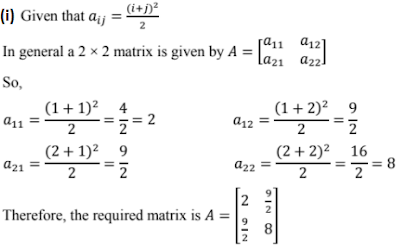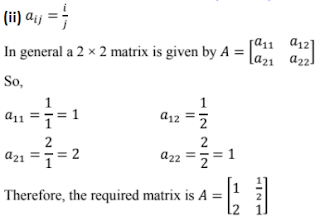5. Construct a 3 × 4 matrix, whose elements are given by:
(i) aij = 1/2 |-3 + j|
(ii) aij = 2i - j

(i) aij = 1/2 |-3 + j|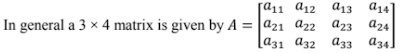a11 = 1/2|−3 × 1 + 1| = 1/2|−3 + 1| = 1
a12 = 1/2|−3 × 1 + 2| = 1/2|−3 + 2| = 1/2
a13 = 1/2|−3 × 1 + 3| = 1/2|−3 + 3| = 0
a14 = 1/2|−3 × 1 + 4| = 1/2|−3 + 4| = 1/2

a21 = 1/2|−3 × 2 + 1| = 1/2|−6 + 1| = 5/2
a22 = 1/2|−3 × 2 + 2| = 1/2|−6 + 2| = 2
a23 = 1/2|−3 × 2 + 3| = 1/2|−6 + 3| = 3/2
a24 = 1/2|−3 × 2 + 4| = 1/2|−6 + 4| = 1

a31 = 1/2|−3 × 3 + 1| = 1/2|−9 + 1| = 4
a32 = 1/2|−3 × 3 + 2| = 1/2|−9 + 2| = 7/2
a33 = 1/2|−3 × 3 + 3| = 1/2|−9 + 3| = 3
a34 = 1/2|−3 × 3 + 4| = 1/2|−9 + 4| = 5/2(ii) aij = 2i - j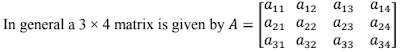a11 = 2 × 1 − 1 = 2 −1 = 1
a12 = 2 × 1 − 2 = 2 −2 = 0
a13 = 2 × 1 − 3 = 2 −3 = -1
a14 = 2 × 1 − 4 = 2 −4 = -2

a21 = 2 × 2 − 1 = 4 −1 = 3
a22 = 2 × 2 − 2 = 4 −2 = 2
a23 = 2 × 2 − 3 = 4 −3 = 1
a24 = 2 × 2 − 4 = 4 −4 = 0

a31 = 2 × 3 − 1 = 6 −1 = 5
a32 = 2 × 3 − 2 = 6 −2 = 4
a33 = 2 × 3 − 3 = 6 −3 = 3
a34 = 2 × 3 − 4 = 6 −4 = 2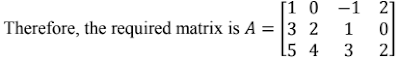6. Find the values of x, y and z from the following equations:(i)As the given matrices are equal, their corresponding elements are also equal.
Comparing the corresponding elements, we get:
x = 1, y = 4, and z = 3

(ii)As the given matrices are equal, their corresponding elements are also equal.
Comparing the corresponding elements, we
get: x + y = 6, xy = 8, 5 + z = 5
Now, 5 + z = 5 ⇒ z = 0
we know that:
(x − y)2 = (x + y)2 − 4xy
⇒ (x − y)2 = 36 − 32 = 4
⇒ x − y = ±2
Now, when x − y = 2 and x + y = 6, we get x = 4 and y = 2
When x − y = − 2 and x + y = 6, we get x = 2 and y = 4
∴ x = 4, y = 2, and z = 0 or x = 2, y = 4, and z = 0

(iii)As the two matrices are equal, their corresponding elements are also equal.
Comparing the corresponding elements, we get:
x + y + z = 9 ... (1)
x + z = 5 ........ (2)
y + z = 7 ........ (3)
From (1) and (2), we have:
y + 5 = 9
⇒ y = 4
Then, from (3), we have:
4 + z = 7
⇒ z = 3
∴ x + z = 5
⇒ x = 2
∴ x = 2, y = 4 and z = 3.

7. Find the value of a, b, c and d from the equation: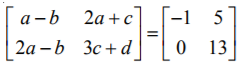As the two matrices are equal, their corresponding elements are also equal.
Comparing the corresponding elements, we get:
a − b = −1 ...... (1)
2a − b = 0 ...... (2)
2a + c = 5 ....... (3)
3c + d = 13 ..... (4)
From (2), we have:
b = 2a
Then, from (1), we have:
a − 2a = −1 ⇒ a = 1 ⇒ b = 2
Now, from (3), we have:
2 ×1 + c = 5 ⇒ c = 3
From (4) we have:
3 × 3 + d = 13
⇒ 9 + d = 13 ⇒ d = 4
∴ a = 1, b = 2, c = 3 and d = 4.

Page No. 65

8. A = [aij]mｘn is a square matrix, if
(A) m < n
(B) m > n
(C) m = n
(D) None of these

It is known that a given matrix is said to be a square matrix if the number of rows is equal to the number of columns.
Therefore, A =[aij]mｘn is a square matrix, if m = n.

9. Which of the given values of x and y make the following pair of matrices equal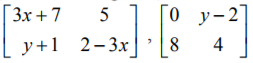(A) x = -1/3, y = 7
(B) Not possible to find
(C) y = 7, x = -2/3
(D) x = -1/3, y = -2/3

It is given that,Equating the corresponding elements, we get:
3x + 7 = 0 ⇒ x = -7/3 and 5 = y − 2 ⇒ y = 7
y + 1 = 8 ⇒ y = 7 and 2 − 3x = 4 ⇒ x = -2/3
We find that on comparing the corresponding elements of the two matrices, we get two different values of x, which is not possible.
Hence, it is not possible to find the values of x and y for which the given matrices are equal.

10. The number of all possible matrices of order 3 × 3 with each entry 0 or 1 is:
(A) 27
(B) 18
(C) 81
(D) 512